EnchantedLearning.com is a user-supported site.
As a bonus, site members have access to a banner-ad-free version of the site, with print-friendly pages.

 EnchantedLearning.comTemperature and Thermometers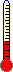Math Activities
Temperature
Temperature is how hot or cold something is. Temperature is really a measure of how fast the atoms and molecules that make up a substance are moving (this movement is sub-microscopic; you cannot see it).

Thermometers Used to Measure Temperature
A thermometer measures the temperature of something, showing how hot or cold it is. There are many different types of thermometers that measure the temperature of different things. We use thermometers to measure the temperature of the air, our bodies, food, and many other things.

There are analog and digital thermometers. An analog thermometer shows the temperature on a scale or dial; a digital thermometer shows the temperature as a number. The higher then number above zero, the higher the temperature.

Temperature Scales
Temperature Fahrenheit Celsius Kelvin
Water Boils 212°F 100°C 373K
Water Freezes 32°F 0°C 273K
Normal Human Body Temperature 98.6°F 37°C 310K
Room Temperature 68°F 20°C 293K
Absolute Zero
(the lowest temperature possible, when molecules have the lowest possible energy)
-459.69°F -273.16°C 0K
Temperature is measured in many different scales, including Fahrenheit, Celsius (or Centigrade), and Kelvin scales. The units of the Celsius and Fahrenheit scales are called degrees; the units of the Kelvin scale are called kelvins. The symbol for degree is °. A change of one degree of temperature Celsius is the same as a change of one kelvin; a change of one degree Fahrenheit is a smaller change in temperature. Each temperature scale is useful for diffferent things.

Fahrenheit is a measure of temperature that is abbreviated F. Water freezes at 32°F and boils at 212°F. This scale was developed by Gabriel Daniel Fahrenheit in 1714. The Fahrenheit scale is used in the USA but not in many other countries. The degrees in Fahrenheit are smaller than those in Celsius, making weather-related temperatures more easily written (without having to use a decimal point).

Celsius (also called Centigrade) is a measure of temperature that is abbreviated C. Water freezes at 0°C and boils at 100°C. This scale was developed by Anders Celsius in 1742.

Kelvin is a measure of temperature that is abbreviated K. Kelvin is a temperature scale designed so that 0K is defined as absolute zero, and the size of one unit is the same as the size of one degree Celsius. [Absolute zero is a hypothetical temperature at which all molecular movement stops. All actual temperatures are above absolute zero. Absolute zero would occur at -273.16°C, -459.69°F, or 0 K.] Water freezes at 273.16K; water boils at 373.16K. Many scientists use this scale (because all the temperatures are positive, making calculations simpler) or the Celsius scale. This temperature scale was designed by Lord Kelvin (William Thomson).

Conversion Formulas
If you know the temperature in one temperature scale you can use a formula to figure out what it is equivalent to in other scales. The conversion formulas are in the table that follows.

 Celsius to Fahrenheit (and Fahrenheit to Celsius): F = 1.8C + 32 C= (F - 32)/1.8 (A degree Celsius is 1.8 times bigger than one degree Fahrenheit.) Kelvin to Celsius (and Celsius to Kelvin): C = K - 273 K = C + 273 (Converting between Celsius and Kelvin is easy because the size of a degree Celsius is the same as the size of a kelvin.)

Activities and Worksheets on Temperature and Thermometers
Writing Worksheets on Temperature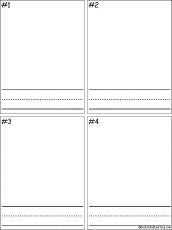Cold Things: Draw Four Things That are Cold in TemperatureDraw four items that are cold in temperature. Below each item, write its name.Hot Things: Draw Four Things That are Hot in TemperatureDraw four items that are hot in temperature. Below each item, write its name.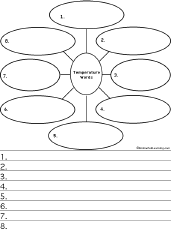Write Eight Temperature WordsThink of and write eight words related to temperature. Then, for each word, write a sentence containing the word. Sample answers: sweltering, glacial, warm, icy, freezing, chilly, cool, nippy.Cold Things: Write Eight Things That are Cold in TemperatureWrite eight things that are cold in temperature. Sample answers: igloo, ice cream, ice cube, freezer, Antarctica, Pluto, glacier, iceberg.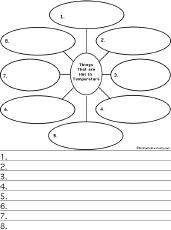Hot Things: Write Eight Things That are Hot in TemperatureWrite eight things that are hot in temperature. Sample answers: campfire, oven, hot cocoa, furnace, star, radiator, molten metal, summer day.Temperature Questions #1:Printable WorksheetOn this worksheet, the student writes about the coldest and hottest things they can think of, and the best and worst things about very hot and very cold days. Go to a pdf version of the worksheet.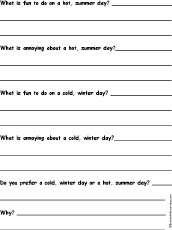Temperature Questions #2:Printable WorksheetOn this worksheet, the student writes about fun things to do on hot and cold days, annoying aspects of hot and cold days, and whether the student prefers very hot or very cold days. Go to a pdf version of the worksheet.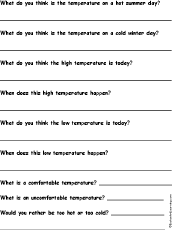Temperature Questions #3:Printable WorksheetOn this worksheet, the student writes about hot, cold, and comfortable temperatures. Go to a pdf version of the worksheet.

Thermometer Worksheets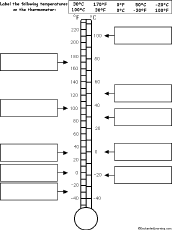Label the Thermometer #1:Printable WorksheetA printable worksheet on which the student labels a thermometer, noting the freezing and boiling points of water, room temperature, body temperature, and the point at which the Fahrenheit and Celsius scales are the same. Or go to the answers. Go to a pdf version.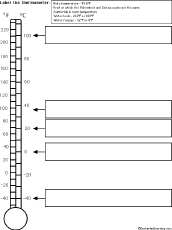Label the Thermometer #2:Printable WorksheetA printable worksheet on which the student labels a thermometer in degrees Fahrenheit and Celsius. Or go to the answers. Go to a pdf version.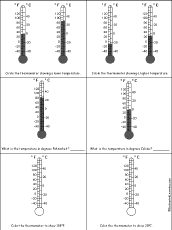Thermometer Questions #1:Printable WorksheetA printable worksheet on temperature and thermometers. Circle the thermometer showing the higher (or lower) temperature, What is the temperature in degrees Fahrenheit (or Celsius)?, and Color the thermometers in degrees Fahrenheit or Celsius. Or go to the answers. Go to a pdf version.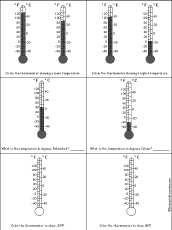Thermometer Questions #2:Printable WorksheetA printable worksheet on temperature and thermometers. Circle the thermometer showing the higher (or lower) temperature, What is the temperature in degrees Fahrenheit (or Celsius)?, and Color the thermometers in degrees Fahrenheit or Celsius. Or go to the answers. Go to a pdf version.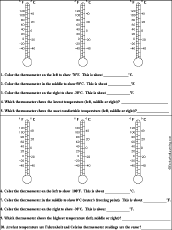Compare Thermometers #1:Printable WorksheetA printable activity worksheet on temperature and thermometers, with thermometers to color and compare, and questions to answer. Or go to the answers. Go to a pdf version.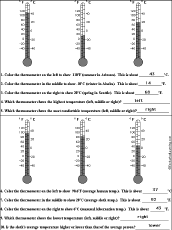Compare Thermometers #2:Printable WorksheetA printable activity worksheet on temperature and thermometers, with thermometers to color and compare, and questions to answer. Or go to the answers. Go to a pdf version.

Temperature Word ProblemsFahrenheit Temperature Word Problems #1:Printable WorksheetA printable activity worksheet of temperature word problems (the problems involve adding or subtracting Fahrenheit temperatures). Or go to the answers. Go to a pdf version.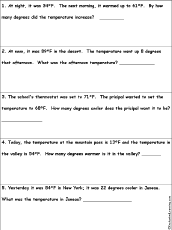Fahrenheit Temperature Word Problems #2:Printable WorksheetA printable activity worksheet of temperature word problems (the problems involve adding or subtracting Fahrenheit temperatures). Or go to the answers. Go to a pdf version.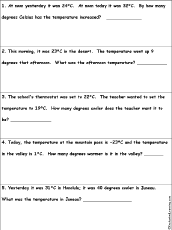Celsius Temperature Word Problems #1:Printable WorksheetA printable activity worksheet of temperature word problems (the problems involve adding or subtracting Celsius temperatures). Or go to the answers. Go to a pdf version.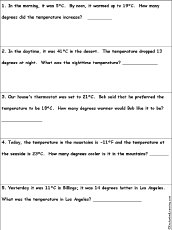Celsius Temperature Word Problems #2:Printable WorksheetA printable activity worksheet of temperature word problems (the problems involve adding or subtracting Celsius temperatures). Or go to the answers. Go to a pdf version.

Charts and Graphs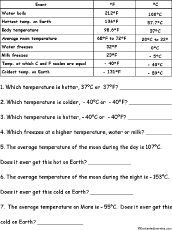Temperature Chart Questions #1:Printable WorksheetA printable activity worksheet in which the student uses a chart of temperatures (in Fahrenheit and Celsius) to answer temperature questions (for example, "Which freezes at a higher temperature, water or milk?"). Or go to the answers. Go to a pdf version.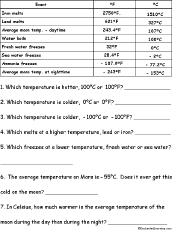Temperature Chart Questions #2:Printable WorksheetA printable activity worksheet in which the student uses a chart of temperatures (in Fahrenheit and Celsius) to answer temperature questions (for example, "Which temperature is hotter, 100°F or 100°C?"). Or go to the answers. Go to a pdf version.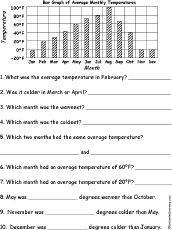Fahrenheit Bar Graph Questions #1:Printable WorksheetA printable activity worksheet in which the student reads a bar graph of Fahrenheit temperatures to answer questions; for example, "What was the average temperature in March?" Or go to the answers. Go to a pdf version of the worksheet.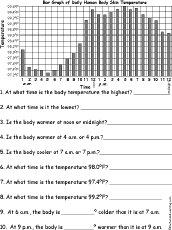Fahrenheit Bar Graph Questions #2:Printable WorksheetA printable activity worksheet in which the student reads a bar graph of daily body temperature in Fahrenheit to answer questions; for example, "At what time is the body temperature the highest?" Or go to the answers. Go to a pdf version of the worksheet.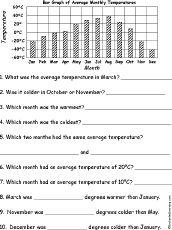Celsius Bar Graph Questions #1:Printable WorksheetA printable activity worksheet in which the student reads a bar graph of Celsius temperatures to answer questions; for example, "What was the average temperature in March?" Or go to the answers. Go to a pdf version of the worksheet.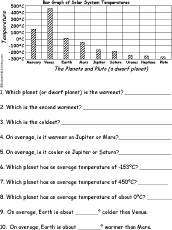Celsius Bar Graph Questions #2:Printable WorksheetA printable activity worksheet in which the student reads a bar graph of the average temperatures of the planets to answer questions, for example, "On average, is it warmer on Jupiter or Mars?" Or go to the answers. Go to a pdf version of the worksheet.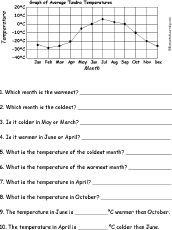Celsius Line Graph Questions #1:Printable WorksheetA printable activity worksheet in which the student reads a line graph of monthly tundra temperatures in Celsius to answer questions; for example, "Which month is the warmest?" Or go to the answers. Go to a pdf version of the worksheet.Celsius Line Graph Questions #2:Printable WorksheetA printable activity worksheet in which the student reads a line graph of atmospheric temperature (in Celsius) by altitude to answer questions; for example, "What is the temperature at 5000m altitude?" Or go to the answers. Go to a pdf version of the worksheet.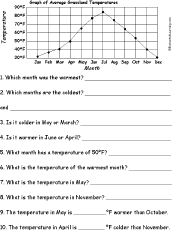Fahrenheit Line Graph Questions #1:Printable WorksheetA printable activity worksheet in which the student reads a line graph of monthly grassland temperatures in Fahrenheit to answer questions; for example, "Which months are the coldest?" Or go to the answers. Go to a pdf version of the worksheet.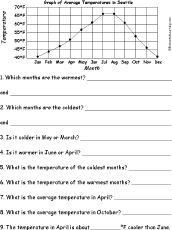Fahrenheit Line Graph Questions #2:Printable WorksheetA printable activity worksheet in which the student reads a line graph of monthly Seattle temperatures in Fahrenheit to answer questions; for example, "What is the temperature of the warmest months?" Or go to the answers. Go to a pdf version of the worksheet.

Measuring TemperatureMeasure Temperatures #1:Printable WorksheetA printable activity worksheet on measuring temperature using a thermometer (the student needs a thermometer to complete this worksheet). The student measures room temperature, the temperature outdoors, and skin temperature. The student then answers questions about these temperatures. Go to a pdf version of the worksheet.Measure Temperatures #2:Printable WorksheetA printable activity worksheet on measuring temperature using a thermometer (the student needs a thermometer to complete this worksheet). The student measures the temperature of ice water, cold tap water and warm tap water. The student then answers questions about these temperatures. Go to a pdf version of the worksheet.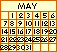Weather Calendar to PrintOn this 13-page calendar (a cover plus 12 monthly pages), the student records local temperatures and other weather occurances.

Converting From Scale to ScaleConvert Temperatures #1:Printable WorksheetA printable activity worksheet on converting temperatures from Fahrenheit to Celsius and vice versa. Or go to the answers. Go to a pdf version of the worksheet.Convert Temperatures #2:Printable WorksheetA printable activity worksheet on converting temperatures from Fahrenheit to Celsius and vice versa. Or go to the answers. Go to a pdf version of the worksheet.

Enchanted Learning®
Over 35,000 Web Pages
Sample Pages for Prospective Subscribers, or click below

 Overview of Site What's New Enchanted Learning Home Monthly Activity Calendar Books to Print Site Index K-3 Crafts K-3 Themes Little ExplorersPicture dictionary PreK/K Activities Rebus Rhymes Stories Writing Cloze Activities Essay Topics Newspaper Writing Activities Parts of Speech Fiction The Test of Time Biology Animal Printouts Biology Label Printouts Biomes Birds Butterflies Dinosaurs Food Chain Human Anatomy Mammals Plants Rainforests Sharks Whales Physical Sciences: K-12 Astronomy The Earth Geology Hurricanes Landforms Oceans Tsunami Volcano Languages Dutch French German Italian Japanese (Romaji) Portuguese Spanish Swedish Geography/History Explorers Flags Geography Inventors US History Other Topics Art and Artists Calendars College Finder Crafts Graphic Organizers Label Me! Printouts Math Music Word Wheels

## Enchanted Learning Search

 Search the Enchanted Learning website for: